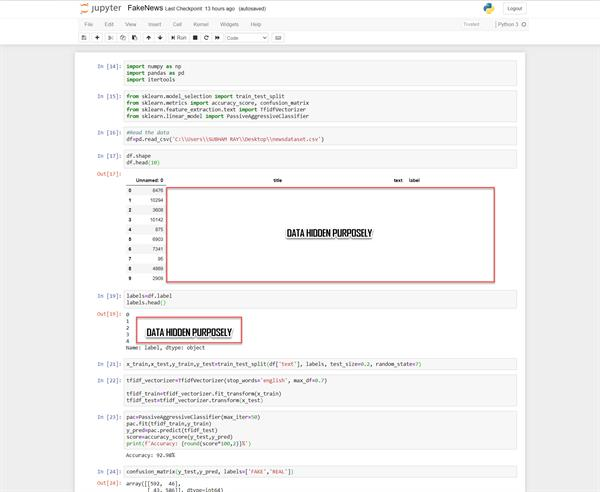Loading, please wait...# How to Detect Fake News using Python

Jul 13, 2020 DataScience, Python, SubhamRay, 6138 Views
DataScience | Python | Detect Fake News

# Detecting Fake News

• What is Python?
• What is Fake News?
• The source code.
• Resources.

### What is the Python Programming Language?

Python Programming language is an interpreted, object-oriented, high-level programming language with dynamic semantics, supporting modules and packages, which encourages program modularity and code reuse. It has the ability to create CSV output for easy data reading in a spreadsheet which alternatively more complicated file outputs that can be ingested by machine learning clusters for computation.

### What is Fake News?

Fake News is Junk news or pseudo News, which usually contains disinformation, intended for misleading information for a particular topic that may contain fabricated headlines to increase readership.

### The Source Code:

• Install libraries with pip
``pip install numpy pandas sklearn​``
• First import the important imports:
``````import numpy as np
import pandas as pd
import itertools​``````
• After that import from sklearn
``````from sklearn.model_selection import train_test_split
from sklearn.metrics import accuracy_score, confusion_matrix
from sklearn.feature_extraction.text import TfidfVectorizer
from sklearn.linear_model import PassiveAggressiveClassifier​``````
• Read the data from the dataset
``````#Read the data
​``````
``````df.shape
• Get the labels from DataFrame from the dataset
``````labels=df.label
• Split Dataset into training and testing sets
``x_train,x_test,y_train,y_test=train_test_split(df['text'], labels, test_size=0.2, random_state=7)​``
• fit and transform the vectorizer on the train set, and transform the vectorizer
``````tfidf_vectorizer=TfidfVectorizer(stop_words='english', max_df=0.7)

tfidf_train=tfidf_vectorizer.fit_transform(x_train)
tfidf_test=tfidf_vectorizer.transform(x_test)​``````
• Initialize the Passive-Aggressive Classifier
``````pac=PassiveAggressiveClassifier(max_iter=50)
pac.fit(tfidf_train,y_train)
y_pred=pac.predict(tfidf_test)
score=accuracy_score(y_test,y_pred)
print(f'Accuracy: {round(score*100,2)}%')​``````
• Apply Confusion Matrix to gain insights
``confusion_matrix(y_test,y_pred, labels=['FAKE','REAL'])​``### Resources

• https://www.python.org/